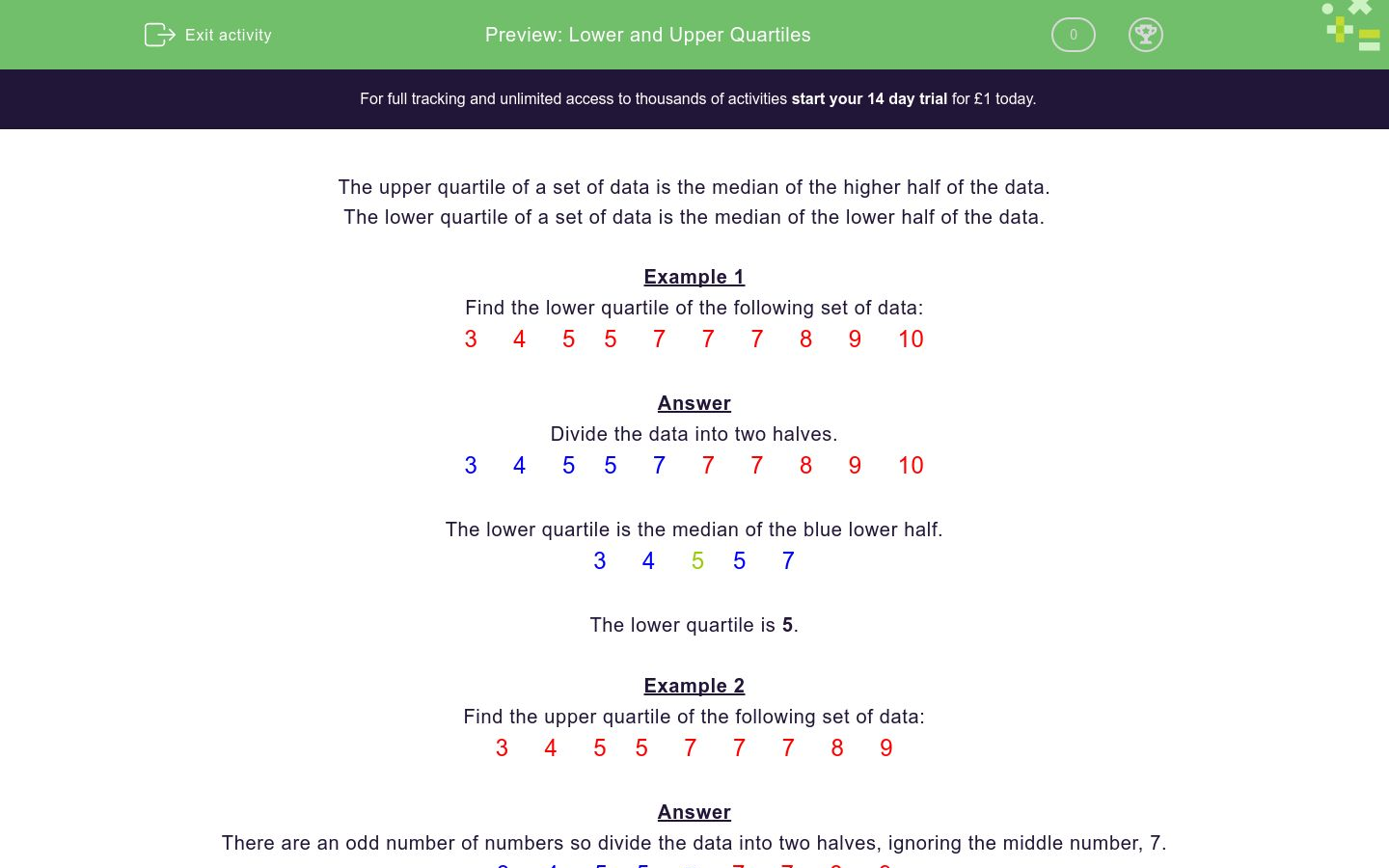# Lower and Upper Quartiles

In this worksheet, students must calculate the upper and lower quartiles of a set of data.Key stage:  KS 4

Curriculum topic:  Statistics

Difficulty level:### QUESTION 1 of 10

The upper quartile of a set of data is the median of the higher half of the data.

The lower quartile of a set of data is the median of the lower half of the data.

Example 1

Find the lower quartile of the following set of data:

3     4     5    5     7     7     7     8     9     10

Divide the data into two halves.

3     4     5    5     7     7     7     8     9     10

The lower quartile is the median of the blue lower half.

3     4     5    5     7

The lower quartile is 5.

Example 2

Find the upper quartile of the following set of data:

3     4     5    5     7     7     7     8     9

There are an odd number of numbers so divide the data into two halves, ignoring the middle number, 7.

3     4     5    5     7     7     7     8     9

The upper quartile is the median of the red higher half.

7     7     8     9

The median here is the mean of 7 and 8.

The upper quartile is.

Find the upper quartile of the following set of data:

3     4     5    5     7     7     7     8     9     10

Find the upper quartile of the following set of data:

5     7     7     7     8     9     10

Find the lower quartile of the following set of data:

5     7     7     7     8     9     10

Find the lower quartile of the following set of data:

5     7     8     9     10

Find the upper quartile of the following set of data:

5     7     8     9     10

Find the upper quartile of the following set of data:

7     8     9     10     12     14

Find the lower quartile of the following set of data:

7     8     9     10     12     14

Find the upper quartile of the following set of data:

15     9     6     12    14        10

Find the lower quartile of the following set of data:

17     9     3     10    14        10

Find the lower quartile of the following set of data:

16     9     1    15     11    14        10    4    3

• Question 1

Find the upper quartile of the following set of data:

3     4     5    5     7     7     7     8     9     10

8
EDDIE SAYS
Median of 7, 7, 8, 9, 10 is 8
• Question 2

Find the upper quartile of the following set of data:

5     7     7     7     8     9     10

9
EDDIE SAYS
Median of 8, 9, 10 is 9
• Question 3

Find the lower quartile of the following set of data:

5     7     7     7     8     9     10

7
EDDIE SAYS
Median of 5, 7, 7 is 7
• Question 4

Find the lower quartile of the following set of data:

5     7     8     9     10

6
EDDIE SAYS
Median of 5 and 7 is 6
• Question 5

Find the upper quartile of the following set of data:

5     7     8     9     10

9.5
EDDIE SAYS
Median of 9 and 10 is 9.5
• Question 6

Find the upper quartile of the following set of data:

7     8     9     10     12     14

12
EDDIE SAYS
Median of 10, 12, 14 is 12
• Question 7

Find the lower quartile of the following set of data:

7     8     9     10     12     14

8
EDDIE SAYS
Median of 7, 8, 9 is 8
• Question 8

Find the upper quartile of the following set of data:

15     9     6     12    14        10

14
EDDIE SAYS
Put the numbers in order first to get 6, 9, 10, 12, 14, 15.
Median of 12, 14, 15 is 14
• Question 9

Find the lower quartile of the following set of data:

17     9     3     10    14        10

9
EDDIE SAYS
Put the numbers in order first to get 3, 9, 10, 10, 14, 17.
Median of 3, 9, 10 is 9
• Question 10

Find the lower quartile of the following set of data:

16     9     1    15     11    14        10    4    3

3.5
EDDIE SAYS
Put the numbers in order first to get 1, 3, 4, 9, 10, 11, 14, 15, 16.
Median of 1, 3, 4, 9 is 3.5
---- OR ----

Sign up for a £1 trial so you can track and measure your child's progress on this activity.

### What is EdPlace?

We're your National Curriculum aligned online education content provider helping each child succeed in English, maths and science from year 1 to GCSE. With an EdPlace account you’ll be able to track and measure progress, helping each child achieve their best. We build confidence and attainment by personalising each child’s learning at a level that suits them.